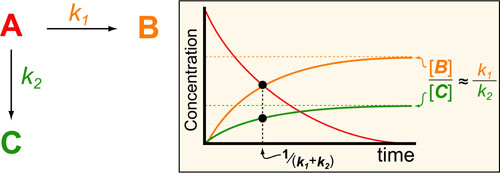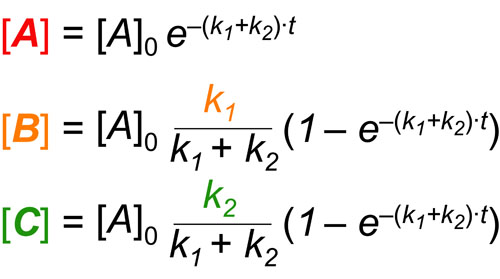# Kinetics #3: Branch Points in Biochemical PathwaysIn a follow up to our posts on Intuiting Enzyme Kinetics and Sequential Biochemical Pathways, we next wanted to consider the kinetic curves branch points in biochemical pathways (or equivalently kinetic competitions). Luckily exact mathematical models exist for competitive first-order processes (see below) and we can use these to develop intuitive rules (figure above) if we consider these pathways to be approximately pseudo-first order (which is often true in the context of biosynthetic pathways).

In the scheme above, k1 and k2 represent the pseudo-first order rate constants for the conversions of A to B and A to C, respectively. In general, the ratio of B and C that forms is equal to the ratio of k1 to k2. In addition, the timescales for all processes are the same.### REFERENCES:

1. Segel, I.H. Enzyme Kinetics: Behavior and Analysis of Rapid Equilibrium and Steady-State Enzyme Systems, Wiley-Interscience, 1993
2. Banford, C.H.; Tipper, C.F.H. Comprehensive Chemical Kinetics. Elsevier, 1969
3. McBane, G.C. Graduate Chemical Kinetics Lecture Notes. OSU875, http://faculty.gvsu.edu/mcbaneg/chem875.pdf
4. Lauffenburger, D.A. Receptors: Models for Binding, Trafficking and Signalling, Oxford University Press 1993.